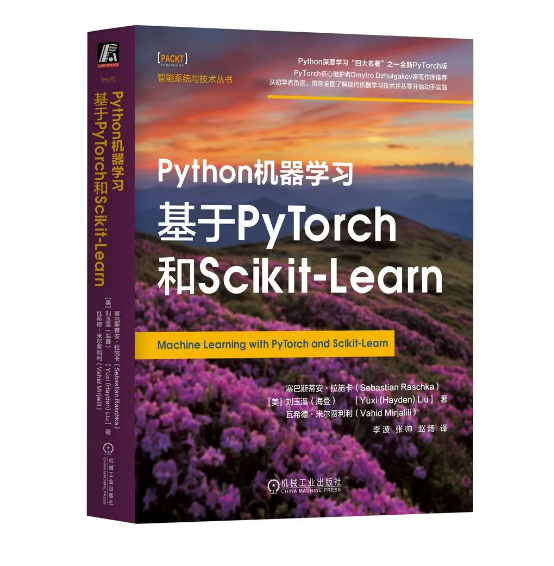## 【玩转pandas系列】数据清洗（文末送书福利）

chatgpt/2023/9/26 12:56:45### 文章目录

• 一、重复值检测
• 二、元素替换
• 1️⃣ 元素替换replace
• 2️⃣ 数据映射map
• 三、修改索引
• 1️⃣ 修改索引名rename
• 2️⃣ 设置索引和重置索引
• 四、数据处理
• 1️⃣ apply与applymap
• 2️⃣ transform
• 五、异常值处理
• 六、抽样聚合函数
• 1️⃣ 抽样
• 2️⃣ 数学函数
• 七、分组聚合
• 🎁 文末福利

# 一、重复值检测

pandas中用于重复值检测的是 `duplicated()` 函数，可以用于检测行或列是否前后重复，返回值是 `bool` 类型。True 表示重复，False 表示不重复。

• `keep`：默认情况下 keep = first ，表示第一行不重复，后面的行参照第一行，取值还有 `last`（认为最后一行不重复），`False`（标记所有重复行）。

• `subset`：只检测某些列是否重复，值是一个列表。

``````import numpy as np
import pandas as pd
``````
``````# 定义生成 dataframe 对象的函数
def make_df(indexs,columns):data = [[str(j)+str(i) for j in columns] for i in indexs]df = pd.DataFrame(data=data,index=indexs,columns=columns)return df
df = make_df(list('123'),list('ABC'))
df
````````````# 让第一行和第二行一样
df.loc['1'] = df.loc['2']
df
````````````df.duplicated()
````````````df.duplicated(keep='first')
``````

``````df.duplicated(keep='last')
````````````df.duplicated(keep=False)
````````````df.duplicated(subset=['B','C'])
``````

# 二、元素替换

## 1️⃣ 元素替换replace

replace 函数用于替换单个的元素。

``````index = ['张三','张三丰','李白','杜甫']
columns = ['Python','Java','H5','UI']
data = np.random.randint(0,100,(4,4))
df = pd.DataFrame(data=data,index=index,columns=columns)
df
````````````df.replace({1:60,11:61})
``````## 2️⃣ 数据映射map

map 一般用于 `Series` 结构，不能用于 `dataframe` ，因此，我们在使用 `map` 来处理 dataframe 对象时，应该取一个 Series （列）。

``````df.map(lambda x:'及格' if x >= 60 else '不及格')
````````````df['Python'].map(lambda x:'及格' if x >= 60 else '不及格')
``````• 1.直接使用 map

``````df.map({4:44,18:81})
``````• 2.使用 lambda 表达式

``````df['Python'].map(lambda x:'及格' if x >= 60 else '不及格')
``````
• 3.使用普通函数

``````# 使用普通函数
def fn(x):if x >= 60:return '及格'return '不及格'
df['UI'].map(fn)
``````# 三、修改索引

## 1️⃣ 修改索引名rename

``````df.rename({'张三':'ZhangSan'})
````````````df.rename({'Python':'派森'},axis=1)
``````

``````df.rename(columns={'Java':'爪哇'})
``````## 2️⃣ 设置索引和重置索引

pandas中 `set_index()` 函数用于将 keys 参数中指定的列索引变为行索引。

``````df.set_index(keys=['Python'])
``````• `keys`：列表类型，用于指定要更改的列。

• `drop`：bool 类型，默认为True，表示列改为行索引后，删除原来的列。

• `append`：bool 列行，默认为False，表示不追加，删除原来的行索引。``````df.columns = ['1','2','3','4']
df
``````# 四、数据处理

`apply、applymap、transform` 都是用于数据处理的函数，其中 applymap 只适用于处理 dataframe ，apply 和 transform 适用于处理 Series 和 DataFrame 。

## 1️⃣ apply与applymap

apply 应用于Series：

``````df['Python'].apply(lambda x: True if x > 60 else False)
``````apply 应用于DataFrame（求每一列的平均值）：

``````df.apply(lambda x:x.mean())
````````````def fn(x):return (x.mean(),x.count())
df.apply(fn,axis=1)
``````applymap 处理 dataframe ：

``````# 处理Series报错
df['Python'].applymap(lambda x:x*10)
````````````# 处理dataframe不报错
df.applymap(lambda x:x*10)
``````## 2️⃣ transform

transform 处理 Series（计算Python列的平方根）：

``````df['Python'].transform(np.sort)
``````transform 处理 DataFrame（平均值大于6就除以6）：

``````def fn(x):if x.mean() > 6:return x / 6return x
df.transform(fn)
``````# 五、异常值处理

• `describe()`

describe函数用于查看 dataframe 对象的列数，平均值，标准差，最小值、最大值、平均值等。

``````# 构建dataframe对象
data = np.random.randint(0,10,(5,3))
df = pd.DataFrame(columns=['NumPy','Pandas','Pytorch'],data=data)
df
````````````df.describe()
````````````df.describe([0.01,0.33,0.99]).T
``````• `info()、std()`

info 函数用于查看数据类型、行列索引、每一列数据非空情况、类型、内存使用情况等。
std() 函数用于求每一列的标准差。

``````df.info()
df.std()
``````
• `drop()`

drop 函数用于删除索引。可以通过 axis 或者 index / columns 控制删除行索引还是列索引。

``````df2.drop(0) # 默认删除行
df2.drop(index=0) # 等价写法
df2.drop('NumPy',axis=1) # 删除列
df2.drop(columns='NumPy') # 等价写法
df2.drop(columns=['NumPy','Pandas']) # 删除多列
df2.drop(columns='NumPy',inplace=True) # 修改原数据
``````
• `unique()`

unique 函数用于去重，只能应用于 Series 对象，不能用于 DataFrame 对象。

``````df['Pytorch'].unique()
``````
• `query()`

query 函数用于查询特定的行。

``````df.query('Pandas == 6') # 查询pandas = 6的行
df.query('Pytorch > 2') # 查询Pytorch > 2的行
df.query('Pandas == 6 and Pytorch > 2' ) # 使用 and 符
n = 3
df.query('Pytorch > @n') # 使用变量
``````
• `sort_values()`

sort_values 函数作用是根据值对行或列进行排序。

``````df.sort_values('Pandas') # 默认列排序
df.sort_values('Pandas',ascending=False) # 降序
df.sort_values(0,axis=1) # 行排序
``````
• `sort_index()`

sort_index 函数用于对索引排序，数字按大小，非数字按 ASCII 码。

``````df.sort_index() # 默认对行索引排序（axis = 0），并且是升序（ascending = True）
df.sort_index(ascending=False) # 降序
df.sort_index(axis = 1,ascending=False) # 对列索引排序，并且降序
``````

# 六、抽样聚合函数

## 1️⃣ 抽样

pandas 中有两种抽样，分别是有放回抽样（可能重复）和无放回抽样（不会重复），使用 `take` 函数抽取，下面使用代码表示。• 无放回抽样
``````df.take([0,2,3,1,4]) # 交换行
````````````df.take([0,2,1],axis=1) # 交换列
````````````df.take(np.random.permutation([0,1,2,3,4])) # 随机交换
``````• 有放回抽样
``````df.take(np.random.randint(0,4,size=5))
``````## 2️⃣ 数学函数

count非空值数量
max / min最大 / 小值
median / mean中位数 / 平均数
sum
value_counts元素出现次数
cumsum累加
cumprod累乘
std标准差
var方差
cov协方差
corr相关系数

# 七、分组聚合

``````df = pd.DataFrame(data={'item':['萝卜','白菜','辣椒','冬瓜','白菜','辣椒','萝卜','萝卜'],'color':['白色','青色','红色','白色','白色','青色','红色','青色'],'weight':[1,0,3,4,5,2,12,3],'price':[0.99,1.5,2,5,4,2,9,2]}
)
df
``````• 1.求出颜色为白色的价格总和
``````# 要得到颜色为白色的所有行，首先要按照颜色进行分组，通过 by 参数指定
df.groupby(by='color')
# 接下来取出价格列（Series类型）
df.groupby(by='color')['price']
# 进行求和
df.groupby(by='color')['price'].sum()
# 最后取出白色
df.groupby(by='color')['price'].sum()['白色']
``````
• 2.分别求出萝卜的总重量和平均价格
``````# （1）
# 首先按照item分类
df.groupby('item')
# 然后取出重量
df.groupby('item')['weight']
# 求和
df.groupby('item')['weight'].sum()
# 再取出萝卜
df.groupby('item')['weight'].sum()['萝卜']
#（2）
# 类似的，得到平均价格
df.groupby('item')['price'].mean()['萝卜']
``````
• 3.使用 merge 合并总重量和平均价格

``````df1 = df.groupby('item')[['weight']].sum()
df2 = df.groupby('item')[['price']].mean()
display(df1,df2)
````````````# 因为两个 df 对象没有相同列，合并时要使用 left / right_index
df1.merge(df2,left_index=True,right_index=True)
``````# 🎁 文末福利`本书是一本全面介绍在PyTorch环境下学习机器学习和深度学习的综合指南`，可以作为初学者的入门教程，也可以作为读者开发机器学习项目时的参考书。

• 探索机器从数据中“学习”的框架、模型和方法。

• 使用Scikit-Learn实现机器学习，使用PyTorch实现深度学习。

• 训练机器学习分类器分类图像、文本等数据。

• 构建和训练神经网络、transformer及图神经网络。

• 探索评估和优化模型的最佳方法。

• 使用回归分析预测连续目标结果。

• 使用情感分析深入地挖掘文本和社交媒体数据。

✳️ 点这里去往：购买链接

🎁 本次送书`1~5`本【阅读量越多，送的越多】

⌛️ 活动时间：截止到2023-08-8 10:00

✳️ 参与方式： 关注博主+三连（点赞、收藏、评论）（切记要点赞+收藏，否则抽奖无效）

🏆 抽奖方式： 评论区随机抽取小伙伴免费送出！！### opencv04-掩膜

opencv04-掩膜 抠图 #include <iostream> #include <opencv2/highgui/highgui.hpp> #include <opencv2/opencv.hpp> #include <vector> #include <array> #include <algorithm>using namespace std; using namespace cv;int main() {str…

### nginx 超时问题 - 解决方法

nginx.conf 配置模板&#xff1a; http {include mime.types;default_type application/octet-stream;sendfile on;keepalive_timeout 120s;server {listen 80;server_name localhost;################################## 1keepalive_timeout 600s;###…